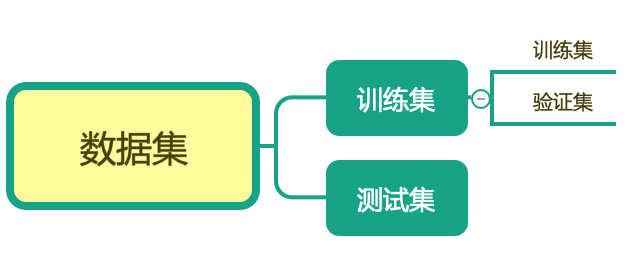2.超参数调优过程

1）将数据集划分为训练集、验证集、测试集

2）选择模型性能评价指标

3）用训练集对模型进行训练

4）在验证集上对模型进行参数搜索，用性能指标来评价参数好坏

5）选出最优参数

3.网络搜索

Grid Search。对模型预设几种超参数组合，选出表现最好的参数，也称为暴力搜索。

交叉验证

cross validation对应的sklearn 的API

sklearn.model-selection.GridSearchCV(estimator, param_grid, scoring=None,                 n_jobs=None, iid='warn', refit=True, cv='warn', verbose=0,                 pre_dispatch='2*n_jobs', error_score='raise-deprecating',                 return_train_score=False)

 参数 含义 estimator 实例化的预估器 param_grid 预估参数，以字典的形式传入("n_neighbors":[1,3,5]) scoring 准确度的评价指标，默认的为None，使用的是score函数 也可以设置其他评价指标，如scoring=‘roc_auc’ n_jobs 并行数，默认为1，-1表示与CPU核数一致 iid 默认为各个样本fold概率分布一致 refit 默认为True：程序会以交叉验证训练集得到最佳参数 cv 几折交叉验证，数据量大的时候，该值稍微小点 verbose 日志容长度，默认为0:不输出训练过程；1:偶尔输出；>1每个子模型都输出 pre_dispatch 指定总共分发的并行任务数。当n_jobs>1时，数据在每个运行点进行复制 error_socre 默认为raise-deprecating:在模型拟合过程中如果产生误差，误差分数会提高 numeric：如果产生误差，fitfailed warning会提高 return_train_score 默认为True：交叉验证结果中包含训练得分

 返回值 含义 best_params 最佳参数 best_score 最佳结果 best_estimator 最佳预估器 cv_results 交叉验证结果

实例

def knn_iris_gscv():    #添加网格搜索和交叉验证    #用knn进行分类    # 从sklearn.datasets中获取到鸢尾花的数据集，使用load_*方法说明是一个比较小的数据集    iris = load_iris()    # 数据分成训练集和测试集    x_train, x_test, y_train, y_test = train_test_split(iris.data, iris.target, test_size=0.2, random_state=10)    # 为防止数据量级差别比较大，所以将数据进行无量纲化，这里采用标准化    standard = StandardScaler()    x_train = standard.fit_transform(x_train)    x_test = standard.fit_transform(x_test)    # 为防止数据量级差别比较大，所以将数据进行无量纲化，这里采用标准化    standard = StandardScaler()    x_train = standard.fit_transform(x_train)    x_test = standard.fit_transform(x_test)    # 传入到knn进行训练，得到模型    classifier = KNeighborsClassifier()    param_dict = {"n_neighbors":[1,3,5,7,9]}   #加入网格搜索和交叉验证    classifier = GridSearchCV(classifier,param_grid=param_dict,cv=4)    classifier.fit(x_train,y_train)    y_predict = classifier.predict(x_test)    print("最佳参数 ：",classifier.best_params_)    print("最佳结果：",classifier.best_score_)    print("最佳预估器：",classifier.best_estimator_)    print("交叉验证结果",classifier.cv_results_)    print("准确度为：", classifier.score(x_test,y_test))    return None

4.随机搜索

Random Search。利用随机数去求函数近似的最优解的方法。

对应的sklearn的API

sklearn.model-selection.RandomizedSearchCV(estimator, param_distributions, n_iter=10, scoring=None,                 n_jobs=None, iid='warn', refit=True,                 cv='warn', verbose=0, pre_dispatch='2*n_jobs',                 random_state=None, error_score='raise-deprecating',                 return_train_score=False）

 参数 含义 estimator 实例化的预估器 param_distributions 字典或列表，需要优化的参数的取值范围 n_iter 抽样参数，默认为10 scoring 准确度的评价指标，默认的为None，使用的是score函数 也可以设置其他评价指标，如scoring=‘roc_auc’ n_jobs 并行数，默认为1，-1表示与CPU核数一致 iid 默认为各个样本fold概率分布一致 refit 默认为True：程序会以交叉验证训练集得到最佳参数 cv 几折交叉验证，数据量大的时候，该值稍微小点 verbose 日志容长度，默认为0:不输出训练过程；1:偶尔输出；>1每个子模型都输出 pre_dispatch 指定总共分发的并行任务数。当n_jobs>1时，数据在每个运行点进行复制 random_state 随机种子。 默认为None：np.random所使用的随机状态实例 int值：随机状态是随机数生成器所使用的种子； 随机实例：随机状态是随机数生成器 error_socre 默认为raise-deprecating:在模型拟合过程中如果产生误差，误差分数会提高 numeric：如果产生误差，fitfailed warning会提高 return_train_score 默认为True：交叉验证结果中包含训练得分

实例

from sklearn.model_selection import RandomizedSearchCVdef knn_iris_random():    # 用knn进行分类    # 从sklearn.datasets中获取到鸢尾花的数据集，使用load_*方法说明是一个比较小的数据集    iris = load_iris()    # 数据分成训练集和测试集    x_train, x_test, y_train, y_test = train_test_split(iris.data, iris.target, test_size=0.2, random_state=10)    # 为防止数据量级差别比较大，所以将数据进行无量纲化，这里采用标准化    standard = StandardScaler()    x_train = standard.fit_transform(x_train)    x_test = standard.fit_transform(x_test)    # 为防止数据量级差别比较大，所以将数据进行无量纲化，这里采用标准化    standard = StandardScaler()    x_train = standard.fit_transform(x_train)    x_test = standard.fit_transform(x_test)    # 传入到knn进行训练，得到模型    classifier = KNeighborsClassifier()    param_dict = {"n_neighbors": range(1,10,2)}    # 加入网格搜索和交叉验证    classifier = RandomizedSearchCV(classifier, param_distributions=param_dict, cv=8)    classifier.fit(x_train, y_train)    y_predict = classifier.predict(x_test)    print("最佳参数 ：", classifier.best_params_)    print("最佳结果：", classifier.best_score_)    print("最佳预估器：", classifier.best_estimator_)    print("交叉验证结果", classifier.cv_results_)    print("准确度为：", classifier.score(x_test, y_test))    return None

5.贝叶斯优化算法

主要思想

（1）首先根据先验分布，假设一个搜索函数；

（2）每一次使用新的采样点来测试目标函数，利用这个信息更新目标函数的先验分布；

（3）算法测试有后验分布给出全局最优解最可能出现的位置点。

• 先验分布

• 后验分布

• 似然估计

两个过程

（1）高斯过程：用以拟合优化目标函数建模，得到其后验分布；

（2）贝叶斯优化：尝试抽样进行样本计算，在局部最优解上不断采样。

• 探索就是在还未取样区域去获取采样点；探索意味着方差高
• 开发就是根据后验分布在最可能出现全局最优解的区域去进行采样；开发意味着均值高

与网格搜索、随机搜索区别

（1）贝叶斯优化采用高斯过程，考虑之前的参数，不断更新先验分布；而网格搜索或随机搜索并没有考虑；

（2）贝叶斯优化迭代次数少，速度快；而网格搜索或随机搜索速度慢，参数过多时，容易导致维度爆炸；

（3）贝叶斯优化针对非凸问题依然稳健；而网格搜索或随机搜索对非凸问题得到局部最优

可以做贝叶斯优化的API

BayesianOptimizationbayesoptskopthyperopt...